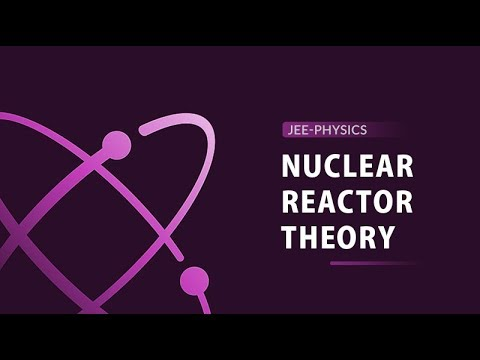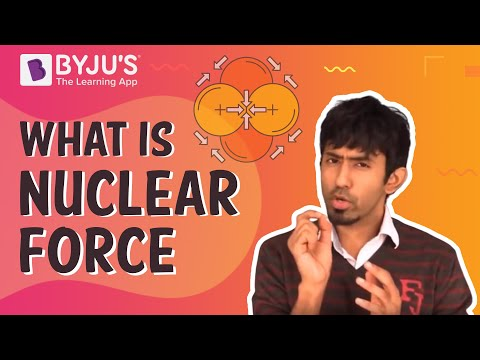# CBSE Class 12 Physics Notes Chapter 13 Nuclei

## Define Nucleus

An atom consists of the nucleus, which is positively charged. The atomic radius is larger than the nucleus’s radius. The mass of an atom is focused on the nucleus. The atom consists of neutrons which have the same mass as protons. The protons and neutrons are bound with each other with a nuclear force. The energies present in nuclear activities are much larger than that of a chemical process.### Isotopes

When the nuclides have the same number of atoms but a different number of neutrons, then it is termed isotopes.

The nuclei of certain elements give out

$$\begin{array}{l}\alpha, \beta,\gamma\end{array}$$
rays and each represents helium nuclei, electrons and electromagnetic radiation, respectively. Radioactivity is the sign of instability of the nuclei.

### Why Fusion Requires High Temperature?

The nuclei, which are light, must have sufficient initial energy to overpower Coulomb’s law between electrical charges having a potential barrier which is only possible in high temperatures.

### Nature of Binding Energy

This shows that nuclear reactions, which are exothermic in nature, are possible in which when a heavy nucleus undergoes fission or two lighter nuclei fuse together to form nuclei with medium mass.

### What Is the Density of Nucleic Matter?

The mass density of an atom does not make it capable of depending upon the size of the nucleus.

#### For more information on Nuclear Reactor Theory and Nuclear Force, watch the below videosTo know more about atomic nuclei, keep visiting BYJU’S website.

### Important Questions

1. If India had a target of producing by 2021 AD, 500,000 MW of electric power, 10% of which was to be obtained from nuclear power plants. Suppose the efficiency of utilization (i.e. conversion to electric energy) of thermal energy produced in a reactor was 50%. How much amount of fissionable uranium would our country need per year by 2021? Take the heat energy per fission of U 235 to be about 300 MeV.
2. If the kinetic energy required for one fusion event =average thermal kinetic energy available with the interacting particles = 2(3kT/2); k = Boltzmann’s constant, T = absolute temperature. What is the kinetic energy needed to overcome the Coulomb repulsion between the two nuclei? Mention the temperature up to which the gas is heated to initiate the reaction. Consider the radius of both deuterium and tritium to be approximately 3.0 fm.
3. $$\begin{array}{l}Fr_{38}^{90}\end{array}$$
half-life is 30 years. Calculate the disintegration rate of 20 mg of the isotope.

Related Topics:

Important Questions on Nuclei

CBSE Class 12 Notes Physics

NCERT Solutions for Class 12 Physics Chapter 13

NCERT Exemplar for Class 12 Physics Chapter 13

Discovery of Neutrons, Isotopes and Isobars

Nuclear Fusion

## Frequently Asked Questions on CBSE Class 12 Physics Notes Chapter 13 Nuclei

Q1

### What is an isotope?

Isotopes are members of a family of an element that all have the same number of protons but different numbers of neutrons.

Q2